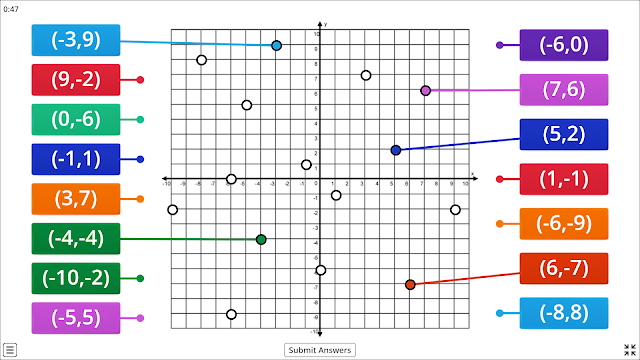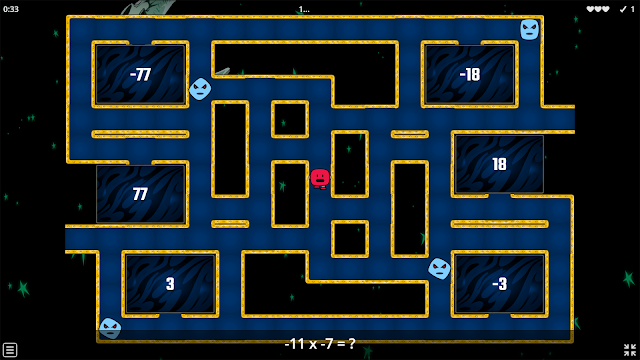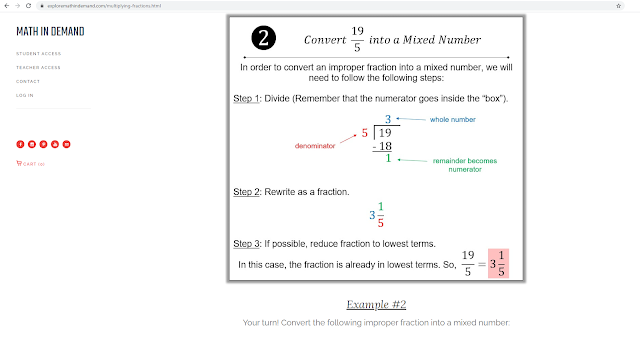### Free Math Resources for Math Notes, Math Games, and Math Online Quizzes

I have been working really hard on my website to provide free online math resources for teachers, parents, and students. Right now my website has various math notes, math games, math videos, and math online quizzes for 6th grade thru 8th grade.Free Math Videos, Math Games, Math Quizzes

On my website, you will see many interactive math games for many different math concepts such as the coordinate plane, order of operations, multiplying integers, dividing integers, least common multiple, greatest common factor, the real number system, slope-intercept form, systems of equations, and so much more!Coordinate Plane Online GameRational Numbers on the Number Line Online GameMultiplying Integers Online GameGreatest Common Factor (GCF) Online GameRationals vs Irrationals Online GameThe Real Number System Online GameSales Tax Online GameSimple Interest Online GameMultiplying Fractions Online GameFree Multiplying Fractions Notes 1Multiplying Fractions Notes 2Volume Video

You can access all of my free resources by clicking HERE

Love my resources and want to buy me a coffee? You can buy me a coffee here...